# HSSlive: Plus One & Plus Two Notes & Solutions for Kerala State Board

## AP Board Class 7 Maths Chapter 10 Construction of Triangles Ex 10.2 Textbook Solutions PDF: Download Andhra Pradesh Board STD 7th Maths Chapter 10 Construction of Triangles Ex 10.2 Book AnswersAP Board Class 7 Maths Chapter 10 Construction of Triangles Ex 10.2 Textbook Solutions PDF: Download Andhra Pradesh Board STD 7th Maths Chapter 10 Construction of Triangles Ex 10.2 Book Answers

## Andhra Pradesh State Board Class 7th Maths Chapter 10 Construction of Triangles Ex 10.2 Books Solutions

 Board AP Board Materials Textbook Solutions/Guide Format DOC/PDF Class 7th Subject Maths Chapters Maths Chapter 10 Construction of Triangles Ex 10.2 Provider Hsslive

2. Click on the Andhra Pradesh Board Class 7th Maths Chapter 10 Construction of Triangles Ex 10.2 Answers.
3. Look for your Andhra Pradesh Board STD 7th Maths Chapter 10 Construction of Triangles Ex 10.2 Textbooks PDF.
4. Now download or read the Andhra Pradesh Board Class 7th Maths Chapter 10 Construction of Triangles Ex 10.2 Textbook Solutions for PDF Free.

## AP Board Class 7th Maths Chapter 10 Construction of Triangles Ex 10.2 Textbooks Solutions with Answer PDF Download

Find below the list of all AP Board Class 7th Maths Chapter 10 Construction of Triangles Ex 10.2 Textbook Solutions for PDF’s for you to download and prepare for the upcoming exams:

Question 1.
Construct ∆ABC with measurements AB = 4.5 cm, BC = 6 cm and ∠B=75°
Given measurements of ∆ABC are AB = 4.5 cm, BC = 6 cm and ∠B = 75°.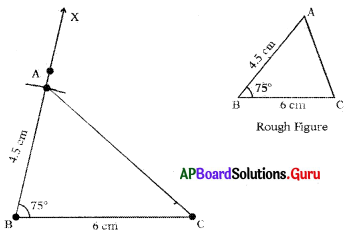Steps of Construction :

1. Draw a rough sketch of triangle and label it with given measurements.
2. Draw a line segment with BC = 6 cm.
3. Draw a ray BX−→− such that ∠CBX = 15°.
4. Draw an arc with centre B and radius 4.5 cm, to intersect BX−→− at point A.
Thus, required ∆ABC is constructed with the given measurements.

Question 2.
Construct an isosceles triangle with measurements DE = 7 cm, EF = 7 cm and ∠E = 60°
Given measurements of ∆DEF are DE = 7 cm, EF = 7 cm and ∠E = 60°.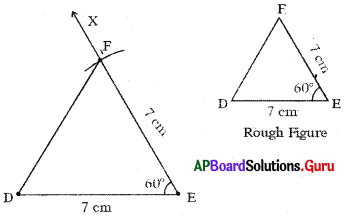Steps of Construction:

1. Draw a rough sketch of triangle and label it with given measurements.
2. Draw a line segment with DE = 7 cm.
3. Draw a ray EX−→− such that ∠DEX = 60°.
4. Draw an arc with centre E and radius 7 cm to intersect EX−→− at point F.
5. Join DF.
Hence, required ∆DEF is constructed with the given measurements.

Question 3.
Draw a triangle with,measurements ∠B = 50°, AB = 3 cm and AC = 4 cm.
Given measurements of a triangle are ∠B = 50°, AB = 3 cm and AC = 4cm..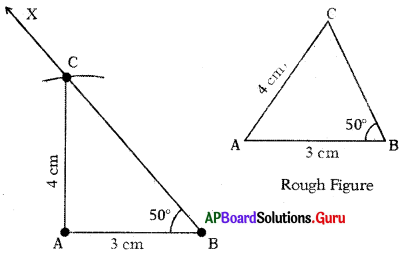Steps of Construction:

1. Draw a rough sketch of triangle and label it with given measurements.
2. Draw a line segment with AB = 3 cm.
3. Draw a ray BX−→− such that ∠ABX = 50°.
4. Draw an arc with centre A and radius 4 cm to intersect BX−→− at point C.
5. Join AC.
Hence, required ∆ABC is constructed with the given measurements.

Question 4.
Construct a right angled triangle in which, XY = 5 cm, XZ = 6 cm and right tingle at X.
Given measurements of ∆XYZ are XY = 5 cm, XZ = 6 cm and ZX = 90° (∠YXZ = 90°)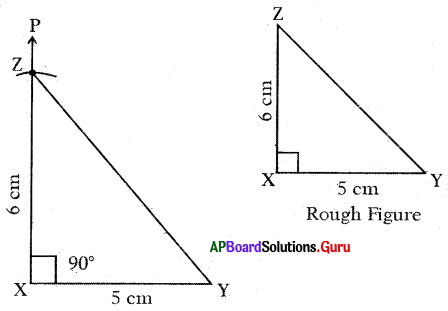Steps of Construction:

1. Draw a rough sketch of triangle arid label it with given measurements.
2. Draw a line segment with XY = 5 cm.
3. Draw a ray XP−→ such that ∠YXP = 90°.
4. Draw an arc with centre X and radius 6 cm to intersect XP at point Z.
5. Join YZ.
Hence, required triangle ∆XYZ is constructed with the given measurements.

Question 5.
Construct a right angled with measurements ABC, ∠B = 90°, AB = 8 cm and AC = 10 cm.
Given measurements of ∆ABC are ∠B = 90°, AB = 8 cm and AC = 10 cm.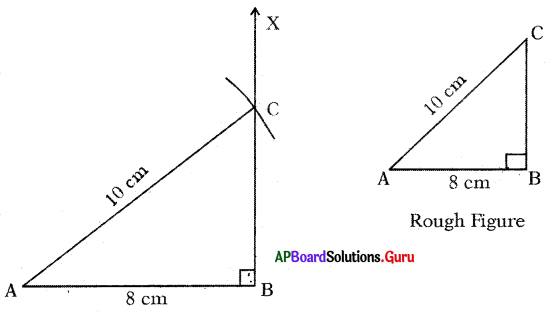Steps of Construction :

1. Draw a rough sketch of triangle and label it with given measurements.
2. Draw a line segment with AB = 8 cm.
3. Draw a ray BX−→− such that ∠ABX = 90°.
4. Draw an arc with centre A and radius 10 cm to intersect BX−→− at point C.
5. Join AC.
Thus, the required ∆ABC is constructed with the given measurements.

## Andhra Pradesh Board Class 7th Maths Chapter 10 Construction of Triangles Ex 10.2 Textbooks for Exam Preparations

Andhra Pradesh Board Class 7th Maths Chapter 10 Construction of Triangles Ex 10.2 Textbook Solutions can be of great help in your Andhra Pradesh Board Class 7th Maths Chapter 10 Construction of Triangles Ex 10.2 exam preparation. The AP Board STD 7th Maths Chapter 10 Construction of Triangles Ex 10.2 Textbooks study material, used with the English medium textbooks, can help you complete the entire Class 7th Maths Chapter 10 Construction of Triangles Ex 10.2 Books State Board syllabus with maximum efficiency.

## FAQs Regarding Andhra Pradesh Board Class 7th Maths Chapter 10 Construction of Triangles Ex 10.2 Textbook Solutions

#### Can we get a Andhra Pradesh State Board Book PDF for all Classes?

Yes you can get Andhra Pradesh Board Text Book PDF for all classes using the links provided in the above article.

## Important Terms

Andhra Pradesh Board Class 7th Maths Chapter 10 Construction of Triangles Ex 10.2, AP Board Class 7th Maths Chapter 10 Construction of Triangles Ex 10.2 Textbooks, Andhra Pradesh State Board Class 7th Maths Chapter 10 Construction of Triangles Ex 10.2, Andhra Pradesh State Board Class 7th Maths Chapter 10 Construction of Triangles Ex 10.2 Textbook solutions, AP Board Class 7th Maths Chapter 10 Construction of Triangles Ex 10.2 Textbooks Solutions, Andhra Pradesh Board STD 7th Maths Chapter 10 Construction of Triangles Ex 10.2, AP Board STD 7th Maths Chapter 10 Construction of Triangles Ex 10.2 Textbooks, Andhra Pradesh State Board STD 7th Maths Chapter 10 Construction of Triangles Ex 10.2, Andhra Pradesh State Board STD 7th Maths Chapter 10 Construction of Triangles Ex 10.2 Textbook solutions, AP Board STD 7th Maths Chapter 10 Construction of Triangles Ex 10.2 Textbooks Solutions,
Share: